# Generate costume shapes

I had this idea - can I build filled costume shapes from simple equation and I could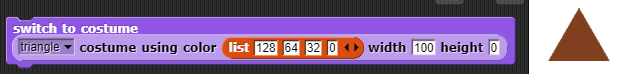Example - Equilateral Triangle (using small values for demo purposes - real block uses 1-100 range)

This gives a list of row widths for a 90/60/30 right-angled triangle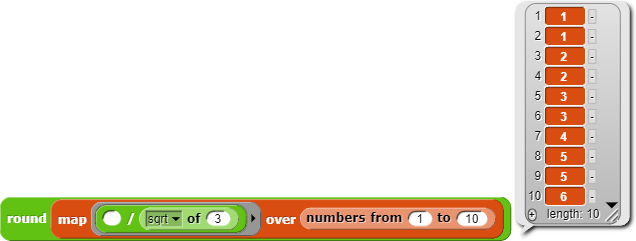This gives a 10 item list with values from 1 to 6 (approx the ratio of a 90/60/30 righ-angle triangle)

Bit of mapping converts this to a 2D matrix

so we can now see the triangle shape

The next bit of mapping joins the right-angled triangle to a reversed copy of itself to give us the full shape

Next stage is to simply flatten the matrix

so that we can then convert each point into a 4 item pixel using a supplied colour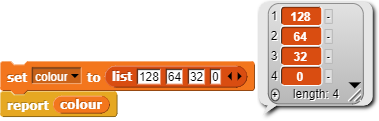And finally convert it to a costume

I've used the same technique to produce most of the other shapes in the custom reporter

e.g. The hexagon is a right-angled triangle appended to a rectangle appended to a reversed copy of the triangle in the horizontal direction to produce a trapezium - then this trapezium is appended to a reversed copy of itself vertically.

The rectangle comes from a suggestion by @jguille2 - make a 1x1 costume and simply scale itThe circle is made using same general technique as the triangle - produce quarter of a circle - add revered copy to get semicircle then add reversed copy of that to give final matrix

ProjectName=costumeFromShape

[edit - the colour list can be replaced by a color reporter from the Colors and Crayons library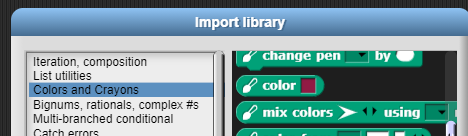Nice program! Although, for the diamond, couldn't you just make a square costume then flip the sprite?

I'd have to do a matrix rotation

[edit
But Snappers can simply create a square and rotate it themselves if that is what you meant]

Nice project!

I'm tempted to try an alternate version that takes a predicate function as input; that predicate function takes two inputs, each in the range [0, 100], and reports True if that pixel should be turned on. That should let you avoid all the rotation/reflection business. I don't have time today but maybe later...

Compare this BJC lab.

I played around with using the BJC lab idea but found it was a bit slower for a circle and felt it would be quite hard to achieve for the pentagon.

But I improved by method by just calculating all the vertical widths in one go and then applying them in one go so my code is smaller

Also added ability to take in colours in other formats while I was on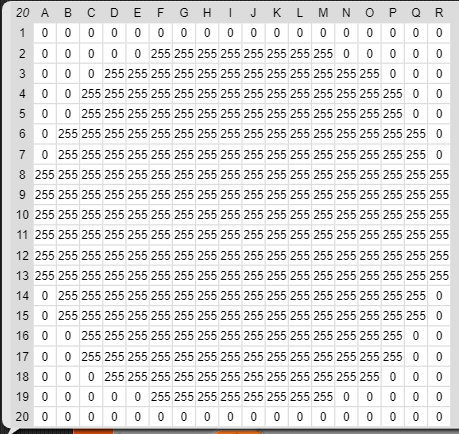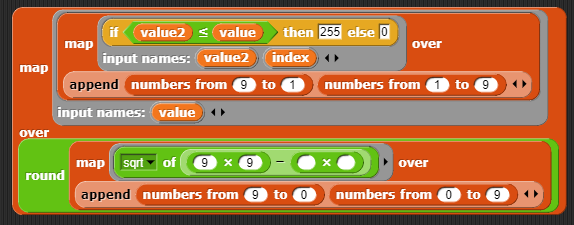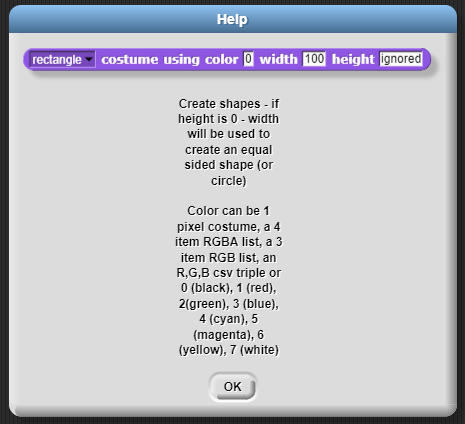ProjectName=costumeFromShape